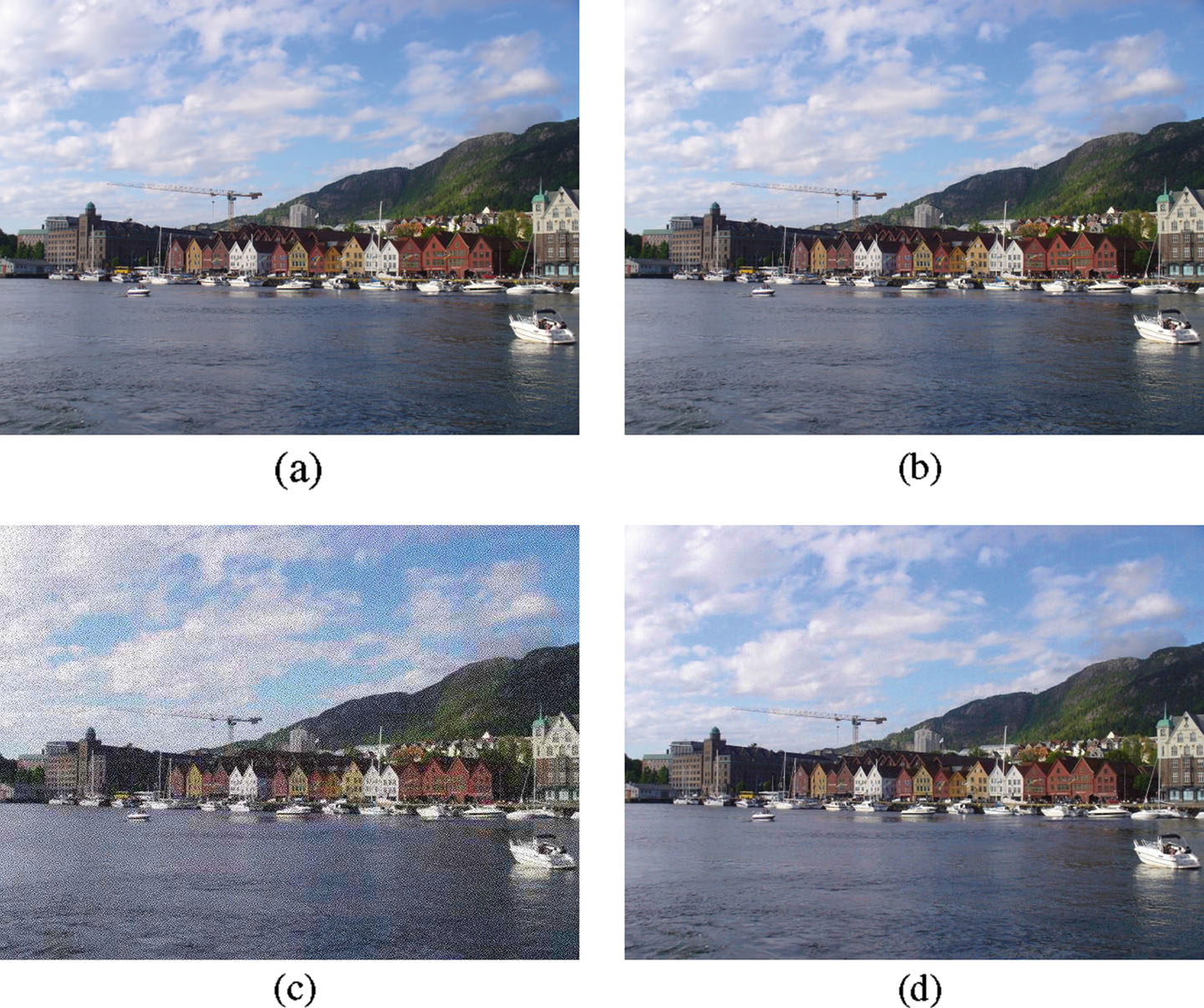Encryption Domain Content-based Image Retrieval and Convolution through a Block-based Transformation Algorithm

Computer Graphics & Multimedia Lab., NTUST

Abstract

### In this paper, we propose a block-based transformation algorithm to achieve the purpose of image content protection. More importantly, under the proposed image content protection framework, image retrieval and image convolution can also be performed directly on the content-protected images. As a consequence, not only secure image storage and communication are accomplished, but also the computation efforts can be fully distributed, thus making it a perfect match for nowadays popular cloud computing technology. Security analyses are conducted to prove that the proposed image encryption scheme offers certain degree of security in both statistical and computational aspects. Although a higher data confidentiality may be reached by adopting traditional cryptographic encryption algorithms, we believe it could be accepted by ordinary users with general image storage needs, since extra functionalities, i.e. content-based image retrieval and image convolution, are provided. Experimental results also demonstrate the decent performance of the proposed encryption domain image retrieval and convolution with acceptable storage overhead.

[Paper]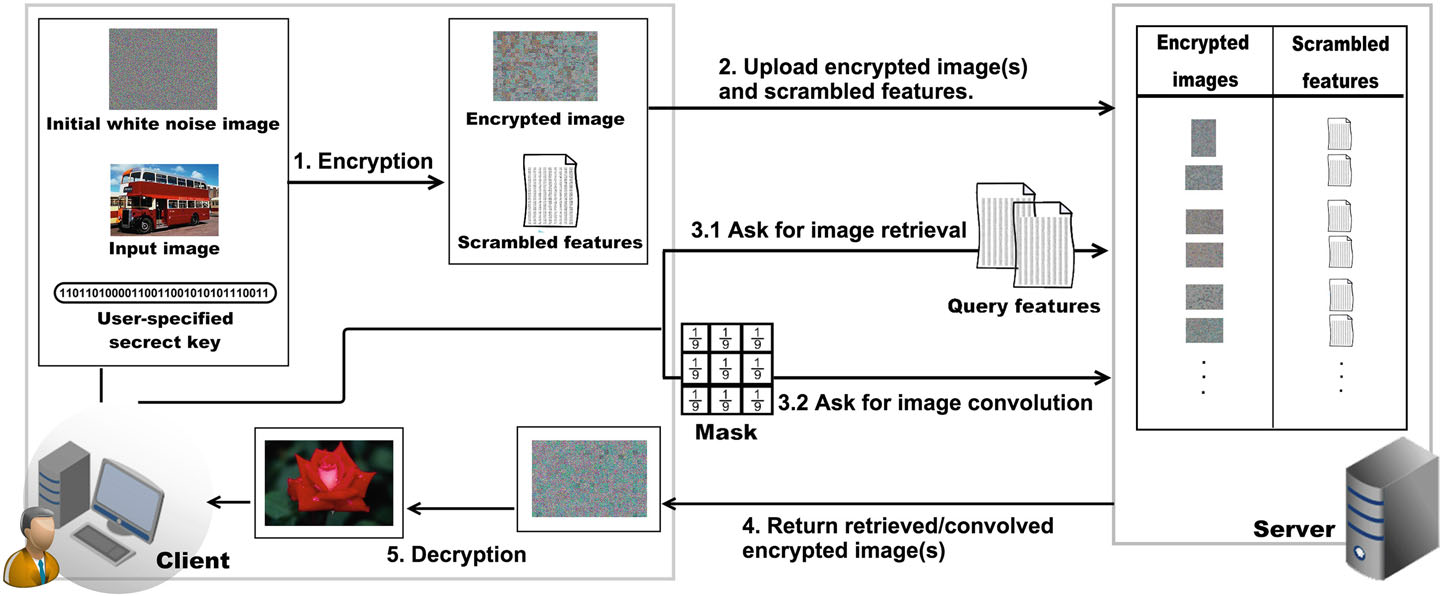### Figure 1: The workflow of the proposed image encryption/decryption scheme.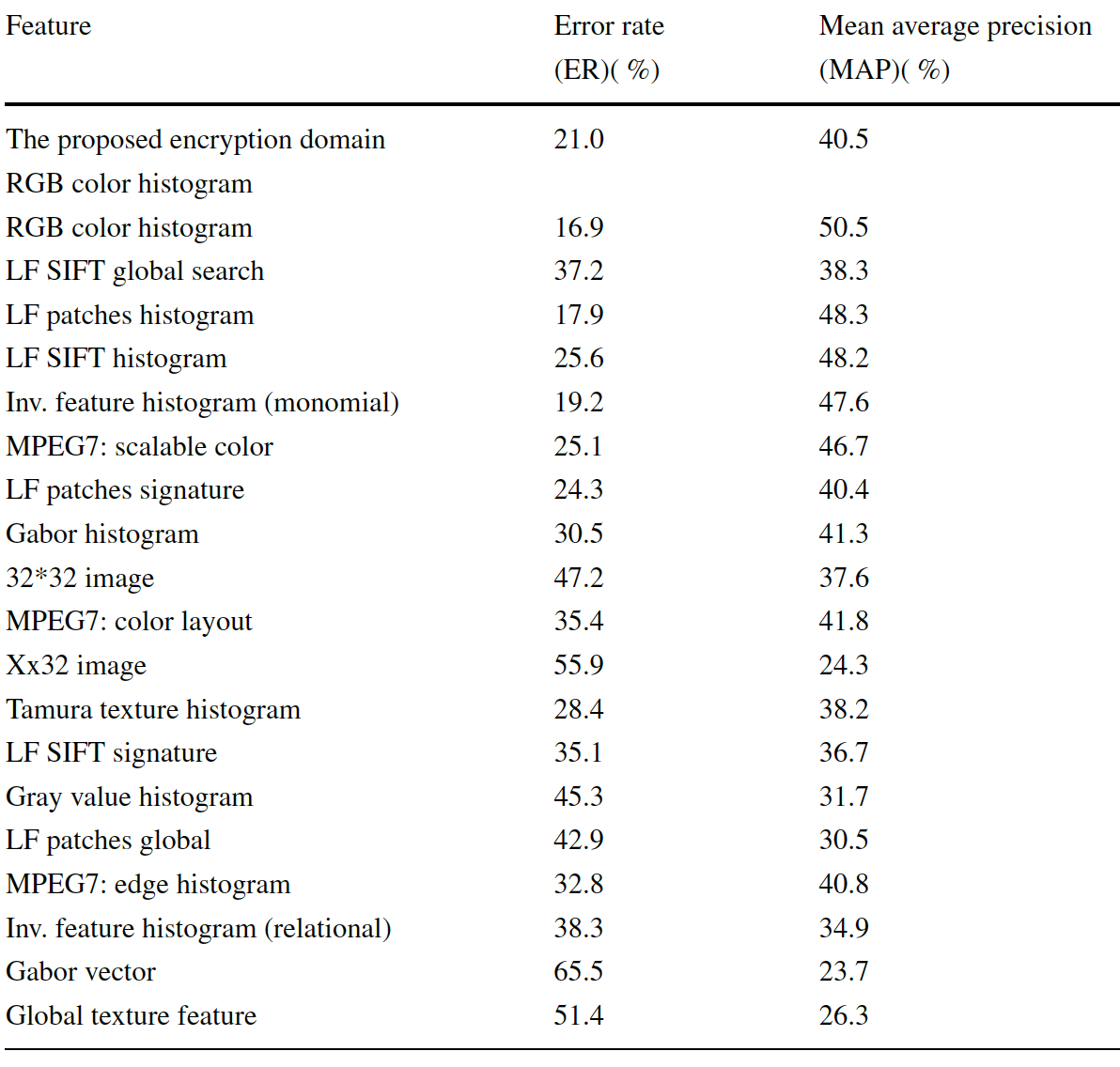### Comparison of the image retrieval performance between the proposed method and the ones listed in [Deselaers et al.'s paper].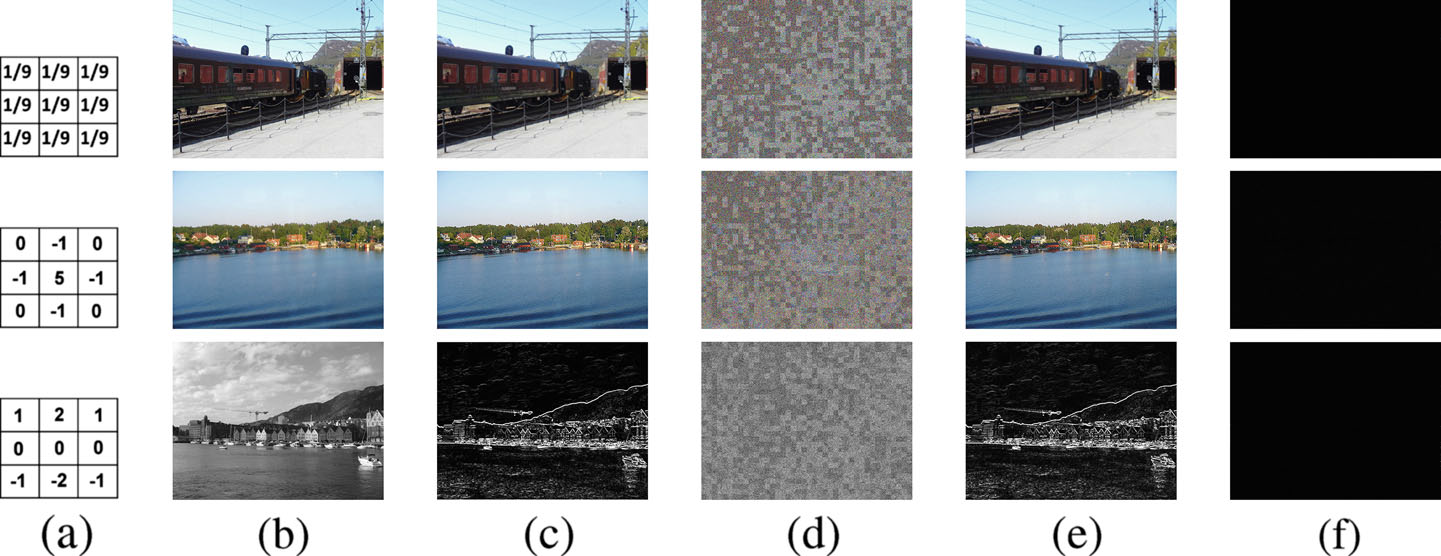### Figure 2: Comparing the results of image convolutions in the plain domain and in the proposed encryption domain. Three basic image processing techniques, blurring, sharpening, and edge detection, are involved and the results are arranged in rows. (a) The convolution kernels used. (b) Input images. (c) Image convolution in the plain domain. (d) The encrypted images. (e) Image convolution applied on (d), and followed by the decryption. (f) The pixel-wise difference between (c) and (e). Note that, in the edge detection example (third row) we only applied the horizontal Sobel operator. Moreover, we did not set a threshold to identify the edge pixels. Therefore, the convolved results, (c) and (e), show the absolute intensity after the convolution rather than the detected edges while intensity values over 255 are set to 255.### Figure 3: An example of the correlation coefficients computed from an original image and its associated encryption. The image is encrypted with permutation block size equals to 16 ? 16 and no overlapped pixels on the sub-block boundaries. And the number of pairs of selected neighboring pixels is 5000. (a) An original image. (b) & (c) The distribution of the adjacent pixels randomly picked from the original image vertically and horizontally, respectively. (d) The encrypted image. (e) & (f) The distribution of the adjacent pixels randomly picked from the encrypted image vertically and horizontally, respectively. g The correlation values of the original image and the encrypted image Also, the averaged correlation values of all the encrypted results of the images in D1 and D2 are listed.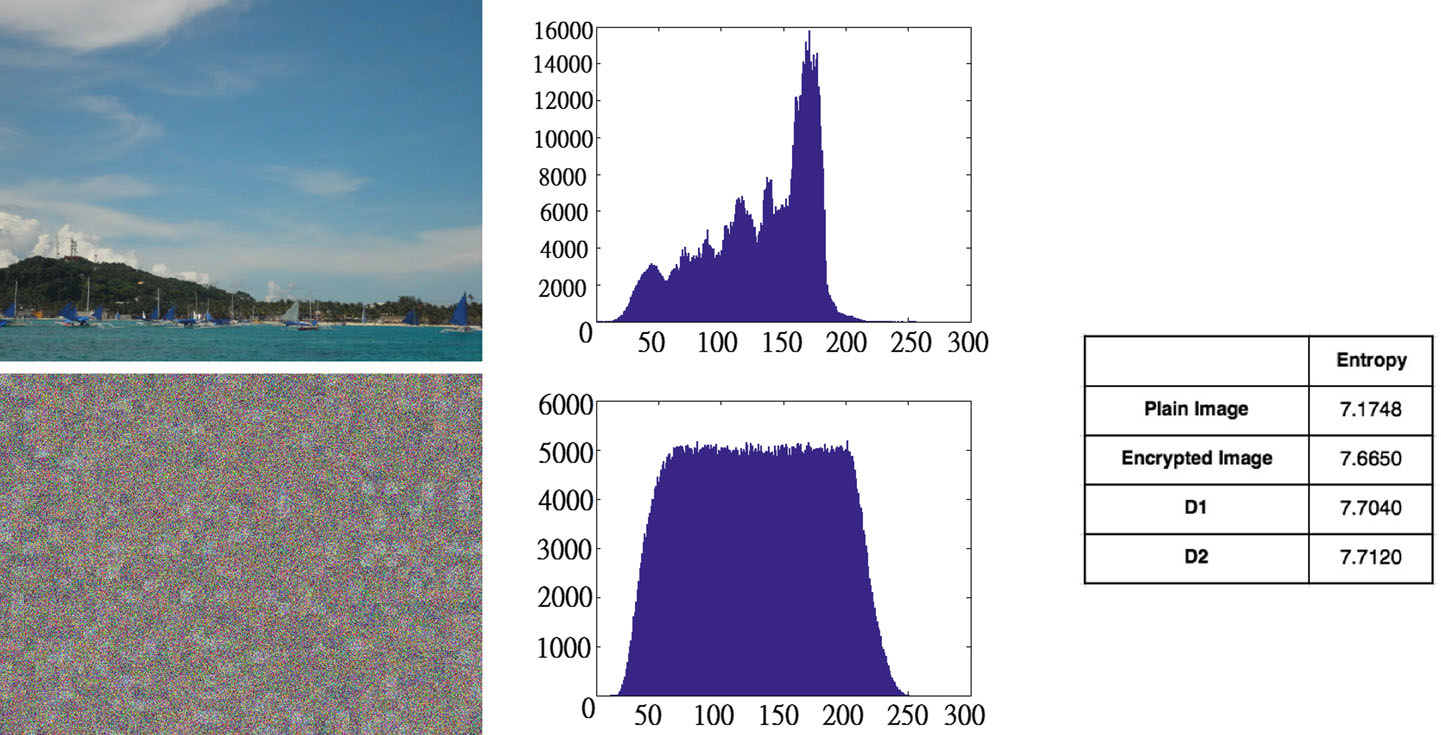### Figure 4: An example of the information entropy calculated from an original image and its associated encryption. The image is encrypted with permutation block size equals to 16 ? 16 and no overlapped pixels on the sub-block boundaries. (a) An original image. (b) The histogram of the pixel values in the original image. (c) The encrypted image. (d) The histogram of the pixel values in the encrypted image. (e) The entropy values of the original image and the encrypted image. Also, the averaged entropy values of all the encrypted results of the images in D1 and D2 are listed.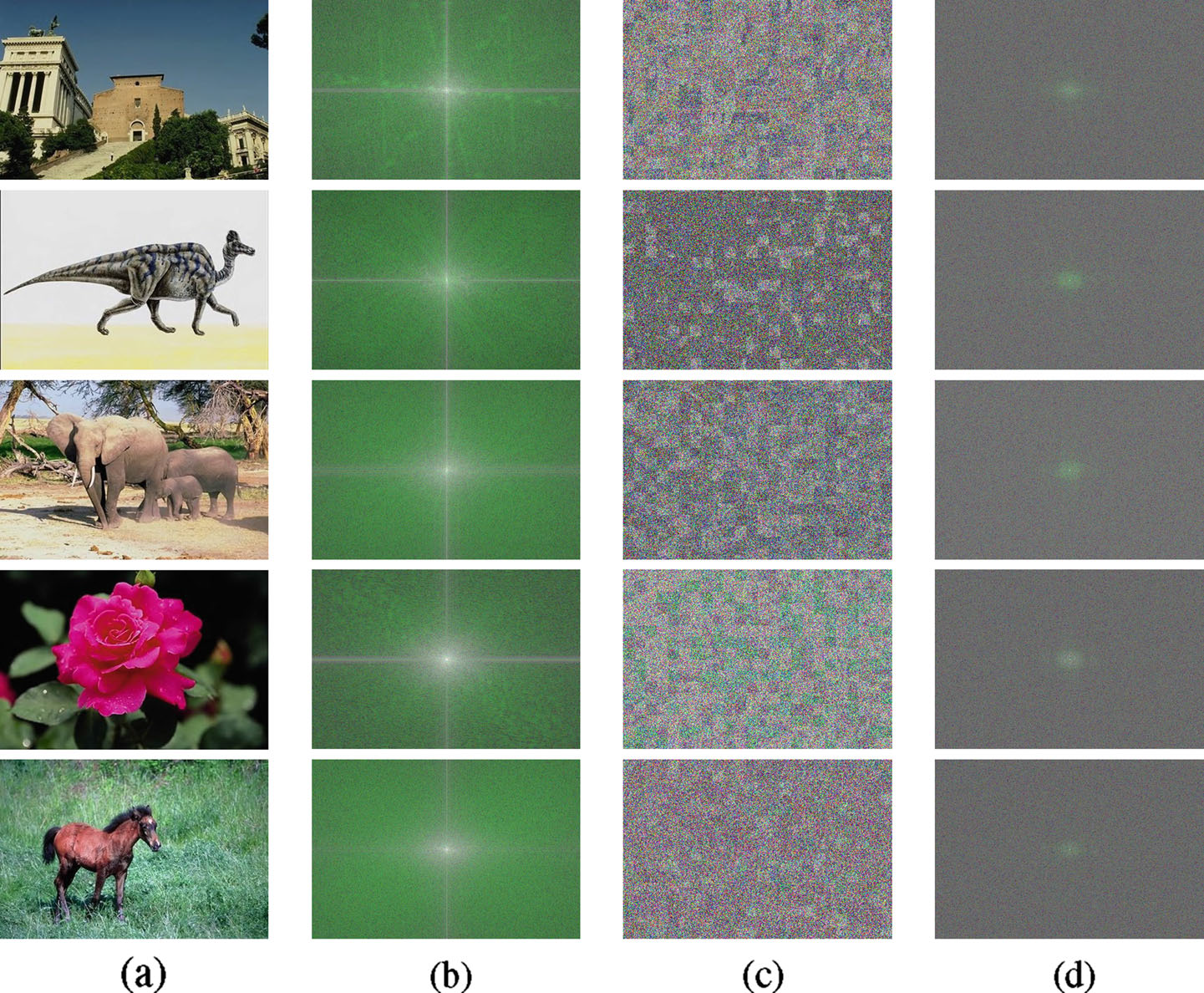### Figure 5: (a) Five randomly chosen images from D1. (b) The FFT spectrums of (a). (c) The corresponding encrypted results of (a). (d) The FFT spectrums of (c).